Trial ends in
JoVE Science Education
Aeronautical Engineering

A subscription to JoVE is required to view this content.
You will only be able to see the first 20 seconds.

Cross Cylindrical Flow
##### Science Education (Aeronautical Engineering)
Airfoil Behavior: Pressure Distribution over a Clark Y-14 Wing
##### Science Education (Aeronautical Engineering)
Turbulence Sphere Method: Evaluating Wind Tunnel Flow Quality

# Cross Cylindrical Flow: Measuring Pressure Distribution and Estimating Drag Coefficients

### Overview

Source: David Guo, College of Engineering, Technology, and Aeronautics (CETA), Southern New Hampshire University (SNHU), Manchester, New Hampshire

The pressure distributions and drag estimations for cross cylindrical flow have been investigated for centuries. By ideal inviscid potential flow theory, the pressure distribution around a cylinder is vertically symmetric. The pressure distribution upstream and downstream of the cylinder is also symmetric, which results in a zero-net drag force. However, experimental results yield very different flow patterns, pressure distributions and drag coefficients. This is because the ideal inviscid potential theory assumes irrotational flow, meaning viscosity is not considered or taken into account when determining the flow pattern. This differs significantly from reality.

In this demonstration, a wind tunnel is utilized to generate a specified airspeed, and a cylinder with 24 ports of pressure is used to collect pressure distribution data. This demonstration illustrates how the pressure of a real fluid flowing around a circular cylinder differs from predicted results based on the potential flow of an idealized fluid. The drag coefficient will also be estimated and compared to the predicted value.

### Principles

The non-dimensional pressure coefficient, Cp, for an arbitrary position in ideal potential flow theory at any angular position, θ, on the surface of a circular cylinder is given by the following the equation:The pressure coefficient Cp is defined as: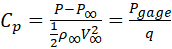where P is the absolute pressure, P is the undisturbed free-stream pressure, Pgage = P − P is the gage pressure, and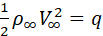is the dynamic pressure, which is based on the free-stream density, ρ, and airspeed, V.

The flow pattern predicted by ideal potential flow theory is shown in Figure 1. The flow is symmetrical, and therefore there is zero net drag force. This is called D'Alembert's Paradox .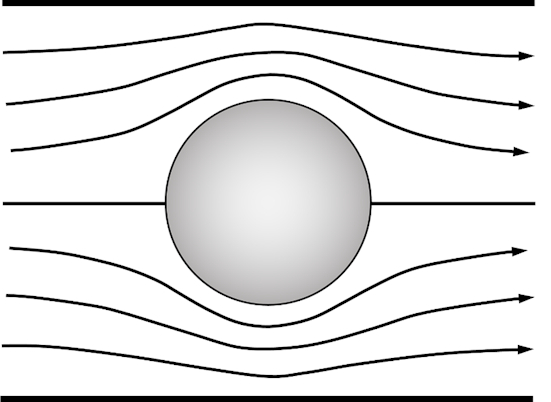Figure 1. Flow pattern of an ideal cross-cylindrical flow in a wind tunnel.

However, a net zero drag force is not expected under real flow conditions. The drag force of a cylinder, FD, per unit length of the cylinder due to pressure differences is given by:The integration is taken along the perimeter of the cylinder.

In this experiment, gage pressure measurements are collected from 24 pressure ports along the cylinder. Then, the above equation can be numerically evaluated using the measured gage pressure as follows:where Pgagei is the gage pressure at the location of θi, θi is the angular position, r is the radius of the cylinder, and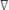θ is the angular distance between adjacent ports, which is 15°. The gage pressure is determined using a manometer panel with 24 independent columns, the gage pressure is determined using the following equation: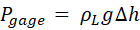where Δh is the height difference of the manometer in reference to the free-stream pressure, ρL is the density of the liquid in the manometer, and g is the acceleration due to gravity. Once the drag force is obtained, the non-dimensional drag coefficient CD can be determined through:where d = 2r is the diameter of the cylinder.

Remembering D'Alembert's Paradox, the drag force is due to the neglected effects of viscosity. First, a boundary layer develops along the cylinder as a result of viscous forces. These viscous forces cause skin-friction drag. Second, the cylinder is a bluff (non-streamlined) object. This creates flow separation and a low-pressure wake behind it and causes a larger drag force due to the pressure differential. Figure 2 displays several typical flow patterns that are observed experimentally. Real flow patterns rely on the Reynolds number, Re, which is defined as: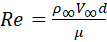where the parameter μ is the dynamic viscosity of the fluid.Figure 2. Various types of flow patterns over a cylinder.

### Procedure

1. Measuring the pressure distribution around a cylinder

1. Remove the top cover of the test section of a wind tunnel, and mount a clean, aluminum cylinder (d = 4 in) with 24 built-in ports on a turntable (Figure 3). Install the cylinder so that port zero is facing upstream (Figure 4a).
2. Replace the top cover, and connect the 24 pressure tubes labeled 0 - 23 to the corresponding ports on the manometer panel. The manometer panel should be filled with colored oil but marked in water in. graduations (Figure 5).
3. Turn on the wind tunnel and run it at 60 mph. Record all 24 pressure measurements by reading the manometer. At this airspeed, the Reynolds number is 1.78 x 105. The expected flow pattern is shown in Figure 2d.
4. Once all measurements have been recorded, turn off the wind tunnel and tape two strings (d = 1 mm) vertically on the cylinder to create the disturbed cylinder. Tape one string between ports 3 and 4 (θ = 52.5°) and the other between ports 20 and 21 (θ = 307.5°). Make sure the ports nearby are not blocked by the tape, as shown in Figure 4b.
5. Turn on the wind tunnel, and repeat step 3. Record all pressure measurements.Figure 3. Gage pressure measurement layout of cross cylindrical flow.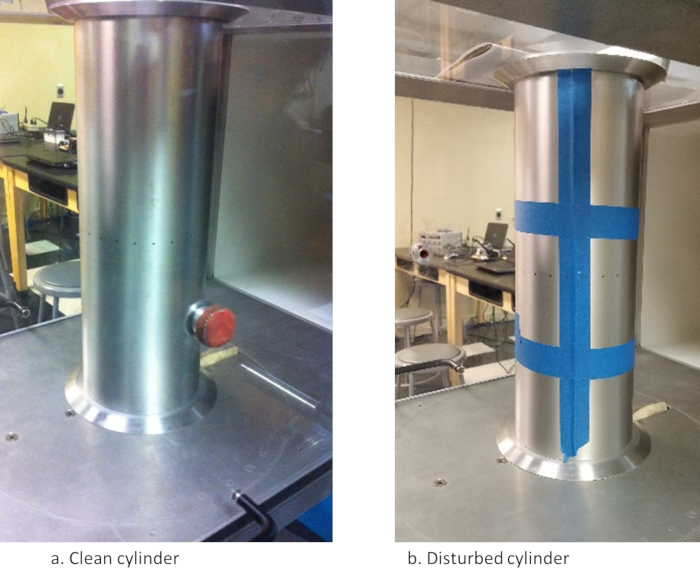Figure 4. Setup of the cylinder in the wind tunnel (pressure ports are in the middle of the cylinder).Figure 5. Manometer panel.

As fluid flows around an object, such as a cylinder, the pressures and velocities close to the object constantly change. According to inviscid potential flow theory, the pressure distribution around a cylinder is symmetric, not only horizontally but also vertically, upstream and downstream of the cylinder. This results in a zero net drag force.

However, experimental results give different flow patterns, pressure distributions and drag coefficients because the inviscid potential theory does not take into account fluid viscosity, which differs greatly from reality.Taking viscosity of the fluid into account, we can further understand real flow patterns around a cylinder.

First, a boundary layer is developed along the cylinder as a result of viscous forces. These viscous forces cause skin friction drag, which is a drag force caused by the friction of the fluid moving across the surface of the object.

Since the cylinder is a bluff body, meaning that it is not streamlined, flow separation occurs and a low pressure wake forms behind the object. This leads to an even bigger form of drag due to a pressure differential.

The characteristics of this flow pattern rely on the Reynolds number. The Reynolds number is a dimensionless number used to describe fluid, and it is a ratio of the inertial forces to the viscous forces. Rho infinity is the density of the fluid, V infinity is the free stream velocity, D is the diameter of the cylinder, and mu is the dynamic viscosity of the fluid.

Below a Reynolds number of about 4, the flow pattern shows very little flow separation behind the cylinder. As the Reynolds number increases, flow separation increases. Below a Reynolds number of about 40, we see a fixed pair of vortices in the wake.

At higher Reynolds number, the vortices shift to a vortex street with a pattern of alternating vortices caused by a process called vortex shedding. At even higher Reynolds number, after the laminar boundary layer has undergone the transition to turbulent, the wake becomes disorganized.

Finally, at very high Reynolds number and turbulent flow, we see the wake become narrower and fully turbulent.

In this lab, we will subject a cylinder with 24 pressure ports to fluid flow in a wind tunnel. We will then use the pressure measurements at each pressure tap to examine the pressure distribution and determine the drag forces on the cylinder.

For this experiment, use an aerodynamics wind tunnel with a test section of 1 ft by 1 ft. Also, obtain an aluminum cylinder with 24 built-in ports for pressure tubes. A manometer panel with 24 columns will also be needed.

To begin, first remove the top cover of the test section. Insert the tubes that connect to the cylinder ports through the slit in the bottom of the test section. Then mount the cylinder on top of the turntable orienting it so that port zero is facing upstream.

Replace the top cover of the test section, and connect the 24 pressure tubes labeled zero through 23 to the corresponding ports of the manometer panel.

Once all of the tubes are properly connected, start the wind tunnel. Increase the wind speed to 60 miles per hour and record all of the 24 pressure measurements by reading the manometer. Now, set the wind speed back to zero and turn off the wind tunnel. Open the test section.

Now, modify the cylinder by securing a 1-mm diameter string vertically between ports 3 and 4, which is equivalent to theta equal to 52.5°. Keep the string as straight as possible while taping it in place. Tape another string between ports 20 and 21, which is theta equal to 307.5°. These strings will disturb the air flow. Use a pin to puncture holes through the blue tape so that the ports can sense the flow pressures.

Then, close the test section. Turn the wind tunnel back on, and increase the wind speed back to 60 miles per hour. Record the 24 pressure measurements using the manometer.

When finished, set the wind speed back to zero and turn off the wind tunnel. Disconnect the tubes from the manometer. Then open the test section and remove the cylinder.

Now, let's interpret the results. First, we can determine the Reynolds number using the free stream velocity, which was 60 miles per hour. The diameter of the cylinder, viscosity and density of the free stream are known. Thus, the Reynolds number is equal to 1.78 x 105.

At this Reynolds number, we can expect a flow pattern as shown, where flow separation occurs and results in a turbulent low pressure wake behind the cylinder. This pressure differential leads to drag.

Now, let's look at our experimental data, in this case for the clean cylinder. Due to symmetry, we will look at only ports 1 through 12. Theta is the angular position of the port, and P-gage is the manometer reading.

First, calculate the non-dimensional pressure coefficient for each port where rho infinity and V infinity are the free stream density and velocity, respectively. Do the same calculation for the disturbed cylinder.

If we plot the experimental results for each cylinder as compared to the ideal, we can see that the stagnation point, or theta equal to zero, the pressure coefficient is at its maximum for both the clean and disturbed cylinders. Before theta equal to 60°, the clean and disturbed cylinders agree well with the ideal data.

After 60°, they deviate from the ideal as they form a low pressure region at the back of the cylinder. If we recall the expected flow pattern, we can see that in the wake region of the flow pattern, we should see turbulent vortices and eddies. This phenomenon corresponds well with the low pressure regions measured for both cylinders.

However, differences between the two arise where the strings were added to the cylinder, where the clean cylinder experiences a lower pressure region in the wake than the disturbed cylinder. This is because the disturbed flow tends to wrap around the cylinder more before the flow separation occurs. The boundary layer, which starts as laminar, transitions to turbulent immediately after the disturbance.

You can see that it wraps around the disturbed cylinder more than the clean cylinder, which is always laminar before the flow separation. Because the disturbed flow has a higher back pressure in the wake, it should have a lower drag force. Let's confirm this hypothesis.

First, calculate drag, FD, as shown using the angular position of each pressure port, the angular distance with adjacent ports, the gage pressure at each port, and the radius of the cylinder. Once we've calculated drag for each cylinder, we can calculate the non-dimensional drag coefficient, CD, for each cylinder.

As expected, the drag coefficient is lower for the disturbed cylinder than the clean cylinder. These results also explain why golf balls are dimpled. The dimples cause turbulent boundary layer flow and therefore lower the drag.

In summary, we learned about the characteristic flow patterns observed at different Reynolds numbers and the transition to turbulent flow. We then subjected cylinders to cross flow in a wind tunnel and measured the pressure distribution along their surfaces to determine the drag forces on each.

### Results

Experimental results for the clean and disturbed cylinder are shown in Tables 1 and 2, respectively. The data can be plotted in a graph of the pressure coefficient, Cp, versus angular position, θ, for ideal and real flow as shown in Figure 6.

 Pressure port # Position angle q (°) Pgage from manometer readings (in. water) Calculated pressure coefficient Cp 0 0 1.7 1.00 1 15 1.4 0.83 2 30 0.0 0.01 3 45 -1.7 -0.98 4 60 -2.7 -1.57 5 75 -3.7 -2.15 6 90 -3.3 -1.92 7 105 -3.0 -1.74 8 120 -3.2 -1.86 9 135 -3.2 -1.86 10 150 -3.3 -1.92 11 165 -3.5 -2.03 12 180 -3.4 -1.97

Table 1. Experimental results for the clean cylinder. Due to symmetry, only data for ports number 0-12 are shown.

 Pressure port # Position angle q (°) Pgage from manometer readings (in. water) Calculated pressure coefficient Cp 0 0 1.8 1.05 1 15 1.6 0.93 2 30 0.6 0.35 3 45 -1.3 -0.73 4 60 -2.9 -1.69 5 75 -4.0 -2.31 6 90 -4.0 -2.33 7 105 -1.7 -0.99 8 120 -1.5 -0.89 9 135 -1.4 -0.84 10 150 -1.4 -0.84 11 165 -1.5 -0.87 12 180 -1.4 -0.84

Table 2. Experimental results for the disturbed cylinder. Due to symmetry, only data for ports number 0-12 are shown.Figure 6. Pressure coefficient distribution, Cp, vs angular position, θ, between ideal and real flow.

At the stagnation point, θ = 0°, Cp reaches its maximum value of Cp = 1. For θ < 60°, the pressure coefficient distribution is similar for all three curves. This is where the laminar boundary layer flow is attached to the surface of cylinder. For θ > 60°, the two experimental flow patterns deviate from the ideal flow; they form a low-pressure region in the back of the cylinder, which is filled with turbulent vortices and eddies. This is the called the wake region. It is the pressure difference between the front and the back of the cylinder that causes the large drag that is observed in cross cylindrical flow.

Despite the similarity in flow patterns between the clean cylinder and disturbed cylinder, there are also differences. The disturbed flow tends to wrap around the cylinder more prior to flow separation, and it also has a higher back pressure. This causes less drag, which is verified by the drag calculations. This occurs because the laminar flow in the front of the cylinder has a tendency to flow straight and it is difficult for the flow to wrap around the cylinder. For the disturbed cylinder, the flow immediately transitions into turbulent flow and thus can wrap around the cylinder more than the clean cylinder.

 Flow Configurations Drag coefficient, CD 1. Clean cylinder 1.68 2. Disturbed cylinder 0.78

Table 4. Drag coefficient, CD (Reynolds number Re = 1.78 x 105).

The drag coefficient CD for clean cylinder at 60-mph airspeed or Re = 178,000 has been evaluated experimentally and is approximately 1.5 , which is close to the value of 1.68 that was obtained in this experiment for a clean cylinder.

From previous experimental results , the drag coefficient CD drops at Re = 3 x 105. This is because the transition from laminar flow to turbulent flow occurs naturally even with a smooth cylinder. In the experiment, the turbulent flow transition is observed by simply taping a 1-mm diameter string to the surface of the cylinder. Thus, a lower drag coefficient CD of only 0.78 is obtained for the disturbed cylinder.

### Applications and SummaryX